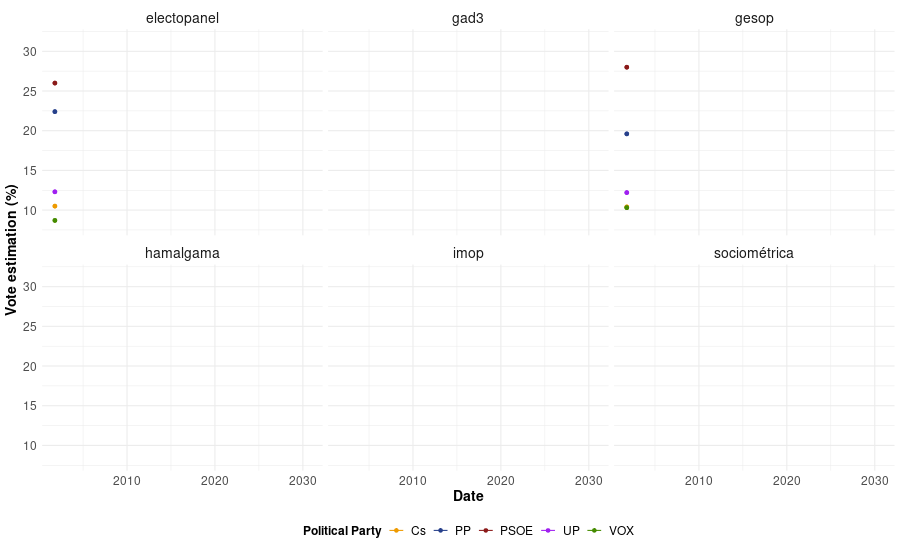# Making some stuff with gganimate

I wanted to make some stuff with the gganimate package since I was aware of the existence of the new API. Yesterday I found the data I was seeking in order to move forward!

El País and its team are making an effort to collect data from many different pollsters and also calibrate some statistical models. The methodology is also explained in the previous link.

My goal here was just focused on trying gganimate, so here is the reproducible example (and the result):

library(readr)
library(tidyr)
library(ggplot2)
library(gifski)
library(gganimate)
library(dplyr)

gga <-
tst %>%
select(date, house, PP, PSOE, UP, Cs, VOX) %>%
gather(key = partido, voto, PP, PSOE, UP, Cs, VOX) %>%
mutate(date = lubridate::as_date(date)) %>%
filter(!is.na(date) & house %in% c('electopanel', 'sociométrica', 'imop',
ggplot(aes(x = date, y = voto, color = partido)) +
geom_line() +
geom_point() +
theme_minimal() +
scale_color_manual(values=c("#EE9A00", "#27408B", "#8B1A1A","#A020F0", "#458B00")) +
labs(x = 'Date', y = 'Vote estimation (%)', color = 'Political Party') +
theme(legend.position = "bottom",
axis.text = element_text(size=12),
axis.title = element_text(size=14, face="bold"),
legend.title = element_text(size = 12, face="bold"),
legend.text = element_text(size = 12),
strip.text = element_text(size = 14)) +
facet_wrap(~ house) +
transition_reveal(date) +
gganimate::animate(gga, height = 550, width = 900, nframes = 150)